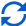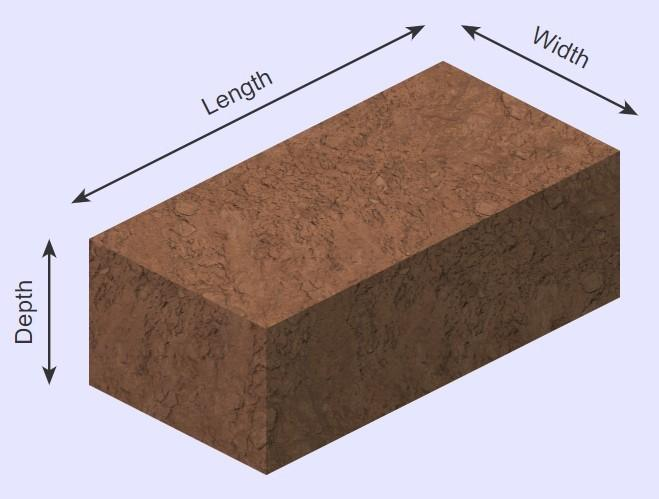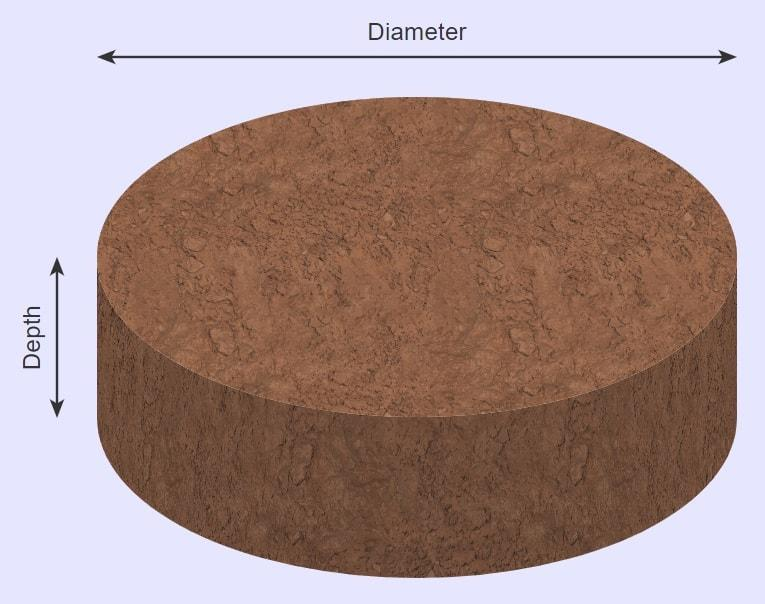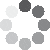Enter parameters Rectangular area
Enter parameters
LENGTH, WIDTH & DEPTH
Length:
ft
• inches (in)
• feet (ft)
• yards (yd)
• centimeters (cm)
• meters (m)
Width:
ft
• inches (in)
• feet (ft)
• yards (yd)
• centimeters (cm)
• meters (m)
Depth:
in
• inches (in)
• feet (ft)
• yards (yd)
• centimeters (cm)
• meters (m)
Bag size:
ft³
• cubic inches (in³)
• cubic feet (ft³)
• cubic yards (yd³)
• cubic centimeters (cm³)
• cubic meters ()
Density:
110 - Topsoil lbs/ft³
• Topsoil - 110 lbs/ft³
• Custom
Price per unit of mass:
t
• pounds (lb)
• US short tons (t)
• imperial-long tons (long t)
• kilograms (kg)
Price per unit of volume:
yd³
• cubic yards (yd³)
• cubic feet (ft³)
• cubic inches (in³)
• cubic centimeters (cm³)
• cubic meters ()Result
Area:
0
ft²
• square feet (ft²)
• square inches (in²)
• square yards (yd²)
• square miles (mi²)
• acres (ac)
• square centimeters (cm²)
• square meters ()
Volume:
0
yd³
• cubic yards (yd³)
• cubic feet (ft³)
• cubic inches (in³)
• cubic centimeters (cm³)
• cubic meters ()
Weight:
0
t
• pounds (lb)
• US short tons (t)
• imperial-long tons (long t)
• kilograms (kg)
Cost:
$Bags: 0 Enter parameters Diameter: ft • inches (in) • feet (ft) • yards (yd) • centimeters (cm) • meters (m) Depth: in • inches (in) • feet (ft) • yards (yd) • centimeters (cm) • meters (m) Bag size: ft³ • cubic inches (in³) • cubic feet (ft³) • cubic yards (yd³) • cubic centimeters (cm³) • cubic meters () Density: 110 - Topsoil lbs/ft³ • Topsoil - 110 lbs/ft³ • Custom Price per unit of mass: t • pounds (lb) • US short tons (t) • imperial-long tons (long t) • kilograms (kg) Price per unit of volume: yd³ • cubic yards (yd³) • cubic feet (ft³) • cubic inches (in³) • cubic centimeters (cm³) • cubic meters ()Result Area: 0 ft² • square feet (ft²) • square inches (in²) • square yards (yd²) • square miles (mi²) • acres (ac) • square centimeters (cm²) • square meters () Volume: 0 yd³ • cubic yards (yd³) • cubic feet (ft³) • cubic inches (in³) • cubic centimeters (cm³) • cubic meters () Weight: 0 t • pounds (lb) • US short tons (t) • imperial-long tons (long t) • kilograms (kg) Cost:$
Bags:
0(1 votes, average: 5.00 out of 5)Loading...
Similar Calculators:
Real-time graphics. Make the calculations and see the changes.
Real-time graphics. Make the calculations and see the changes.
Embed
Similar Calculators:When you start any outdoor landscaping project, it’s important that you have a rough idea of how much material you are going to need.

We know this can be tricky, especially when you are trying to estimate this by eye.

That’s why we created our topsoil calculator.

Not only is it able to work out the volume of topsoil you will need, but also the total weight and cost of the project. 💰

Combining these measurements with a little preparation will ensure that you are ready for your next outdoor landscaping project.

Contents:

## Topsoil calculator formula

Using the measurements you provide from your lawn, our calculator first works out the area and volume of the soil required to ensure equal topdressing coverage.

It does this by using the formulae given below:

### Rectangular Garden BedThe total area of the garden bed and volume of the required topsoil is calculated using the formulae:

$$Area = Length \times Width$$

$$Volume = Area \times Depth$$

### Circular Garden BedThe total area of the garden bed and volume of the required topsoil is calculated using the formulae:

$$Area = \pi \times Radius^2$$

$$Volume = Area \times Depth$$

Where

$$Radius = {Diameter \over 2}$$

You can calculate the total amount of the topsoil required for your lawn if you know the density of the topsoil using the formulae:

$$Weight = Volume \times Density$$

Note that standard topsoil has a density of 100 lb/ft³.

## How does the calculator work out the cost?

If you have the price per unit mass (e.g. cost per ton) or price per unit volume (e.g. cost per cubic foot) then the calculator can work out the total cost of the required soil for topdressing your lawn:

$$Cost = Price\,per\,unit\,mass \times Weight$$

or

$$Cost = Price\,per\,unit\,volume \times Volume$$

At this point, you might be wondering…

What if I’m using a custom soil aggregate on top of my lawn?

Well, our topsoil calculator has an option for “custom density”, which you can calculate using the formulae:

$$Density = {Total\,mass\,of\,topsoil \over Total\,volume\,of\,topsoil}$$

In addition, you can calculate the price per unit mass/volume for the custom soil aggregate:

$$Price\,per\,unit\,mass = {Total\,topsoil\,cost \over Total\,topsoil\,weight}$$

$$Price\,per\,unit\,volume = {Total\,topsoil\,cost \over Total\,topsoil\,volume}$$

Anything not clear? 🤔

See below for two fully worked examples using different initial criteria.

Otherwise, simply enter your measurements and appropriate units into our topsoil calculator to ensure your gardening project gets off to the best of starts!

## Example calculations

• Rectangular garden bed area with standard topsoil

Let’s say that I have a rectangular garden bed which measures 5 feet in length and 4 feet in width.

I want to ensure equal topsoil coverage to a depth of 3 inches with standard topsoil (110 lb/ft³).

The cost of the topsoil is $30 per US short ton. I want to know how much topsoil I will need, the weight of the aggregate and the total cost of the project. I enter these measurements into the calculator which would do the following operations in order to work out the total area of the garden bed: $$Bed\,area = Length \times Width = 5\,ft \times 4\,ft = 20\,ft^2$$ and the volume of the topsoil required: $$Volume = Area \times Depth = 20\,ft^2 \times 3\,in = 5\,ft^3$$ Then, the topsoil calculator would work out the total weight: $$Weight = Volume \times Density = 5\,ft^3 \times 110\,lb/ft^3 = 0.275\,t$$ and total cost of the soil: $$Cost = Price\,per\,unit\,mass \times Weight = 30\,/t \times 0.275\,t = 8.25$$ • Circular garden bed area with custom topsoil aggregate and unknown cost per unit mass For the second example imagine I want to cover a circular garden bed, diameter 8 feet, with a custom topsoil aggregate. I can buy 5 cubic yards for$100, which weighs 5000 pounds. I want to ensure equal coverage to a depth of 4 inches.

For the custom topsoil aggregate I can therefore calculate it density (in pounds per cubic yard) and price per unit mass (in dollars per pound):

$$Density = {Mass \over Volume} = {5000\,lb \over 5\,yd^3} = 1000\,lb/yd^3$$

$$Price\,per\,unit\,volume = {Total\,soil\,cost \over Total\,soil\,volume} = {100 \over 5\,yd^3} = 20\,/yd^3$$

I now enter these measurements into the topsoil calculator to work out the garden bed area:

$$Area = \pi × \biggl( {Diameter \over 2} \biggr) ^2 = \pi × \biggl( {8\,ft \over 2} \biggr) ^2 = 50.27\,ft^2$$

and the total volume of the topsoil required:

$$Volume = Area \times Depth = 50.27\,ft^2 \times 4\,in = 16.76\,ft^3$$

Then, the topsoil calculator works out the total weight:

$$Weight = Volume \times Density = 16.76\,ft^3 \times 1000\,lb/yd^3 = 0.31\,t$$

and total cost of the soil:

$$Cost = Price\,per\,unit\,Mass \times Mass = 20\,/yd^3 \times 0.31\,t = 12.41$$

## What if I want to work with different units?

You might be wondering what to do if your units are in different measurements?

Well, our calculator can take care of this for you.

You might have noticed that we switch between units in our examples.

For example, density is given in pounds per cubic yard and volume is given in cubic feet.

Simply select the correct units in the drop down area for each measurement and the calculator will convert them using the following conversions:

$$1\,foot = 12\,inches = 0.33\,yards = 30.48\,centimeters = 0.3048\,meters$$

$$1\,US\,short\,ton = 2000\,pounds = 0.893\,imperial\,long\,ton = 907\,kg$$

## How many bags of topsoil do i need?

What if you want to work out how many bags of topsoil your landscaping project will require?

This can be easily calculated from the weight/volume of a single bag of the topsoil you will purchase using the formulae:

$$Number\,of\,bags\,required = {Weight\,of\,topsoil\,required \over Weight\,of\,a\,single\,bag}$$

or

$$Number\,of\,bags\,required = {Volume\,of\,topsoil\,required \over Volume\,of\,a\,single\,bag}$$

Let’s take the first example in which we required 0.275 US short tons of topsoil.

Imagine we buy soil in 40 pound bags, which is equivalent to 0.02 tons.

Therefore:

$$Number\,of\,bags\,required = {Weight\,of\,topsoil\,required \over Weight\,of\,a\,single\,bag} = {0.275\,t \over 0.02\,t} = 13.75 \rightarrow 14\,bags$$

It’s that simple! 😉

Embed this calculator on your site!Add live graphics
Copied to clipboard! Preview